# Evaluating Formative Measurement Model - Convergent Validity & Collinearity Diagnostics.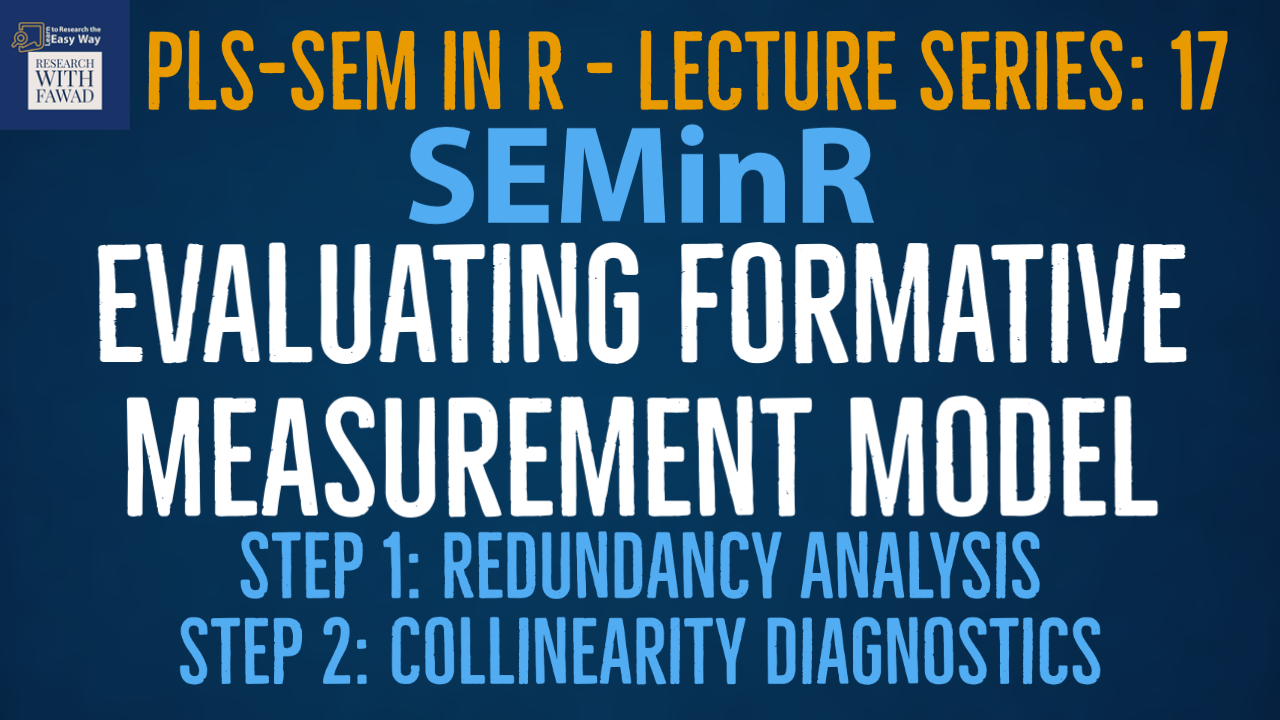### SEMinR Lecture Series

This session is focused on how to perform redundancy analysis and collinearity diagnostics using SEMinR in Cran R.

## Evaluating Formative Measurement Model

• PLS-SEM is the preferred approach when formatively specified constructs are included in the PLS path model (Hair, Risher, Sarstedt, & Ringle, 2019).
• In this part of the series, I discuss the key steps for evaluating formative measurement models (Fig.). Relevant criteria include the assessment of Convergent validity,indicator collinearity, and Statistical significance and relevance of the indicator weights.
• Next, I will introduce key criteria and their thresholds and illustrate their use with an example.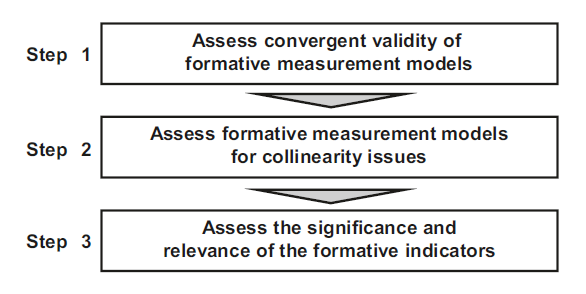## The Example

• The proposed model has three constructs (Vision, Development, and Reward) measured formatively that impact a reflectively measured construct (Collaborative Culture).
• The three constructs are formative constructs, estimated with mode_B, while Collaborative Culture is reflective construct, estimated with mode_A.
• The weights parameter of the composite() function is set by default to mode_A. Thus, when no weights are specified, the construct is estimated as being reflective.
• Alternatively, we can explicitly specify the mode_A setting for reflectively measured constructs or the mode_B setting for formatively measured constructs.
• Once the model is set up, we use the estimate_pls() function to estimate the model, this time specifying the measurement_model and structural_model.
• Finally, we apply the summary() function to the estimated SEMinR model object simple_model and store the output in the summary_simple object.

## The Code

``````library(seminr)
# Create measurement model
simple_mm <- constructs(
composite("Vision", multi_items("VIS", 1:4), weights = mode_B),
composite("Development", multi_items("DEV", 1:7), weights = mode_B),
composite("Rewards", multi_items("RW",1:4), weights = mode_B),
composite("Collaborative Culture", multi_items("CC", 1:6)))
# Create structural model
simple_sm <- relationships(
paths(from = c("Vision","Development","Rewards"), to = "Collaborative Culture"))
# Estimate the model
simple_model <- estimate_pls(data = datas,
measurement_model = simple_mm,
structural_model = simple_sm,
missing = mean_replacement,
missing_value = "-99")
# Summarize the model results
summary_simple <- summary(simple_model)
``````

## Step 1: Convergent Validity

• In formative measurement model evaluation, convergent validity refers to the degree to which the formatively specified construct correlates with an alternative reflectively measured variable(s) of the same concept.
• Originally proposed by Chin (1998), the procedure is referred to as redundancy analysis. To execute this procedure for determining convergent validity, researchers must plan ahead in the research design stage by including an alternative measure of the formatively measured construct in their questionnaire.
• Cheah, Sarstedt, Ringle, Ramayah, and Ting (2018) show that a global single item, which captures the essence of the construct under consideration, is generally sufficient as an alternative measure.
• When the model is based on secondary data, a variable measuring a similar concept would be used (Houston, 2004). Hair et al. (2022) suggest the correlation of the formatively measured construct with the reflectively measured item(s) should be 0.708 or higher, which implies that the construct explains (more than) 50% of the alternative measure’s variance.

## Formative Measurement Model Assessment

• To evaluate the formatively measured constructs, we follow the formative measurement model assessment procedure (Fig.).
• First, we need to examine whether the formatively measured constructs exhibit convergent validity. To do so, we need to carry out a separate redundancy analysis for each construct.
• The original survey contained global single-item measures with generic assessments of the formatively measured construct –Vision. We can use it as measure of the dependent construct in the redundancy analyses (GV).
• Note that when designing a research study that includes formatively measured constructs, you need to include this type of global measure in the survey.
• Figure shows the model set-ups for the redundancy analyses of the four formatively measured constructs in the extended corporate reputation model.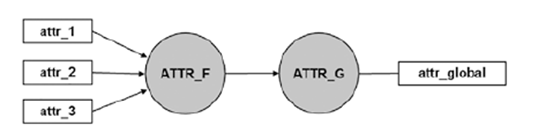## Code for Redundancy Analysis

``````library(seminr)
# Create measurement model
simple_mm <- constructs(
composite("Vision", multi_items("VIS", 1:4), weights = mode_B),
composite("V_G", single_item("GV")))
#Create structural model
simple_sm <- relationships(
paths(from = c("Vision"), to = c("V_G")))
# Estimate the model
Vision_redundancy_pls_model <- estimate_pls(
data = datas,
measurement_model = simple_mm,
structural_model = simple_sm)
#Summarize the model
sum_vis_red_model <- summary(Vision_redundancy_pls_model)
sum_vis_red_model\$paths
``````

## Redundancy Analysis/Convergent Validity

• In order to run the redundancy analysis for a formatively measured construct, it must be linked with an alternative measure of the same concept. When considering the formatively measured construct Vision, the measurement model for the redundancy analysis consists of two constructs: (1) Vision, which is measured by four formative indicators VIS1 to VIS4, and (2) V_G, which is measured by the single item V_G. The structural model consists of a single path from Vision to V_G.
• I then estimate this model using the datas dataset and assign the output to the Vision_redundancy_pls_model object.
• Finally, to identify the path between the two constructs, we need to inspect the sum_vis_red_model\$paths.
• The results for the redundancy analysis of the four formatively measured constructs. For the Vision construct, this analysis yields a path coefficient of 0.818, which is above the recommended threshold of 0.708, thus providing support for the formatively measured construct’s convergent validity.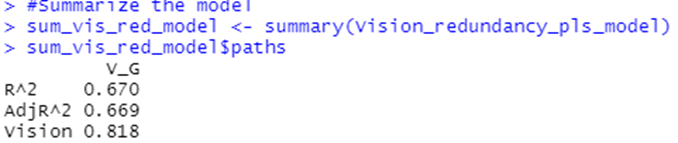## Reference

Hair Jr, J. F., Hult, G. T. M., Ringle, C. M., Sarstedt, M., Danks, N. P., & Ray, S. (2021). Partial Least Squares Structural Equation Modeling (PLS-SEM) Using R: A Workbook.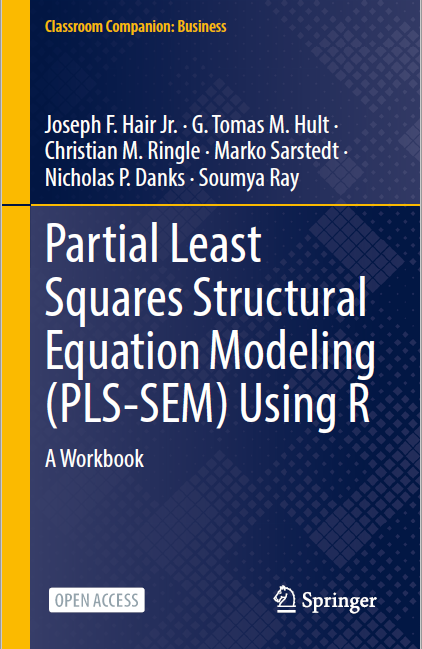The tutorials on SEMinR are based on the mentioned book. The book is open source and available for download under this link.